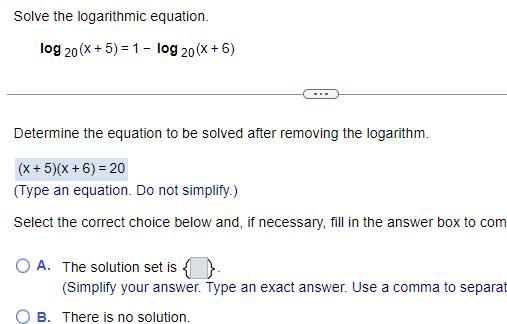Question:

# Solve the logarithmic equation log 20 x 5 1 log 20 X 6

Last updated: 11/21/2023Solve the logarithmic equation log 20 x 5 1 log 20 X 6 Determine the equation to be solved after removing the logarithm x 5 x 6 20 Type an equation Do not simplify Select the correct choice below and if necessary fill in the answer box to com OA The solution set is Simplify your answer Type an exact answer Use a comma to separat B There is no solution